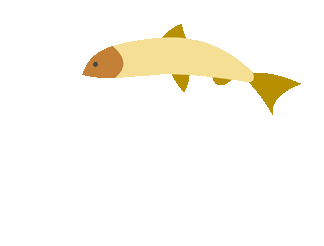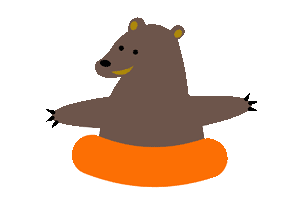top of page## Conversions

##### Fraction and Decimal Conversions (Games)
1. Fruit Shoot

Practice converting fractions and decimals.

2. Puppy Chase

Practice converting decimals to fractions with this multi-player game.

3. Math Playground

Practice converting decimals to fractions with this multi-player game.

4. Death to Decimals and Fractions Game

Convert fractions and decimals.

5. Quia

Practice converting with flashcards, matching, or concentration.

###### Comparing and Ordering Fractions, Decimals, and Mixed Numbers

Practice comparing fractions, decimals, and percents.  Video instructional support is available if you need it.

###### Fraction, Decimal, and Percents on the Number Line
1. Number Line Practice

Practice placing fractions, decimals, and percents on the number line.bottom of page